Open In App
Related Articles
• CBSE Class 9 Maths Revision Notes
• CBSE Class 9 Maths Formulas
• NCERT Solutions for Class 9 Maths
• RD Sharma Class 9 Solutions

# Natural Numbers

Natural Numbers are all positive integers from 1 to infinity and are a component of the number system. Natural numbers are only positive integers, excluding zero, fractions, decimals, or negative numbers, and they are part of real numbers. Natural numbers are also called counting numbers.

## What are Natural Numbers?

Natural numbers or counting numbers are those integers that begin with 1 and go up to infinity. Numbers have applications everywhere, for example, they are used for counting items, representing or trading money, calculating temperature, telling time, and so on. These numbers are referred to as “natural numbers” since they are used to count items. When counting items, it can be 5 glasses, 6 books, 1 bottle, and so on. Therefore, another name for natural numbers is counting numbers. A collection of all whole integers except 0 is referred to as a natural number. These figures play an important role in daily actions and communication.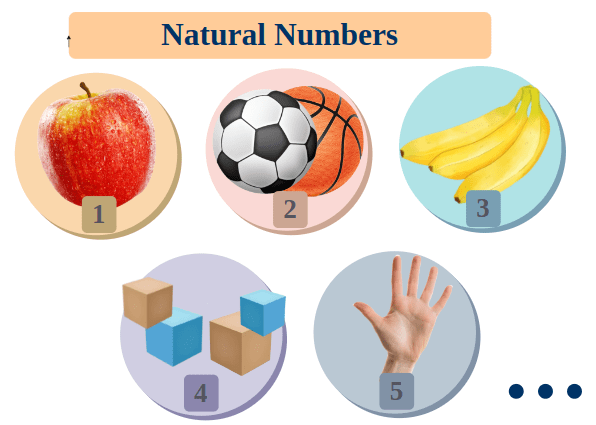## Natural Number Definition

Natural numbers are those that can be counted and are a component of real numbers. Only positive integers, such as 1, 2, 3, 4, 5, 6, etc., are included in the set of natural numbers. Natural numbers start from 1 and go up to ∞.

### Set of Natural Numbers

In mathematics, the set of natural numbers is expressed as 1, 2, 3, … The set of natural numbers is represented by the symbol N. N = {1, 2, 3, 4, 5, … ∞}. A collection of elements is referred to as a set (numbers in this context). The smallest element in N is 1, and the next element in terms of 1 and N for any element in N. 2 is 1 greater than 1, 3 is 1 greater than 2, and so on. The below table explains the different set forms of natural numbers.

Natural numbers are the subset of whole numbers, and whole numbers are the subset of integers. Similarly, integers are the subset of real numbers. The below-given diagram explains the relationship w.r.t. the sets of natural numbers, whole numbers, integers, and real numbers.

## Examples of Natural Numbers

Non-negative integers are also known as natural numbers (all positive integers). 24, 57, 88, 979, 120502, and so forth are a only few instances of examples of Natural Numbers as there are infinitely many natural numbers. Will -4 be a Natural number? No. Since it is a negative integer. Will 3.6 be a natural number? No. Since it is not a positive Integer.

### First 10 Natural Numbers

The first 10 natural numbers are 1, 2, 3, 4, 5, 6, 7, 8, 9, and 10. In the roaster set form, the first 10 natural numbers are represented as,

N = {1, 2, 3, 4, 5, 6, 7, 8, 9, 10}

### Natural Numbers and Whole Numbers

Even natural numbers are those that are divisible by 2 precisely and belong to the set N. So 2,4,6,8,… are examples of even natural numbers. The set of natural even numbers is represented as {2, 4, 6, 8, 10, 12, …}.

### Odd Natural Numbers

Natural numbers that are not divisible by 2 and belong to the set N are known as odd natural numbers. So 1, 3, 5, 7, … are examples of odd natural numbers. The set of natural odd numbers is represented as {1, 3, 5, 7, 9, 11, 13, …}.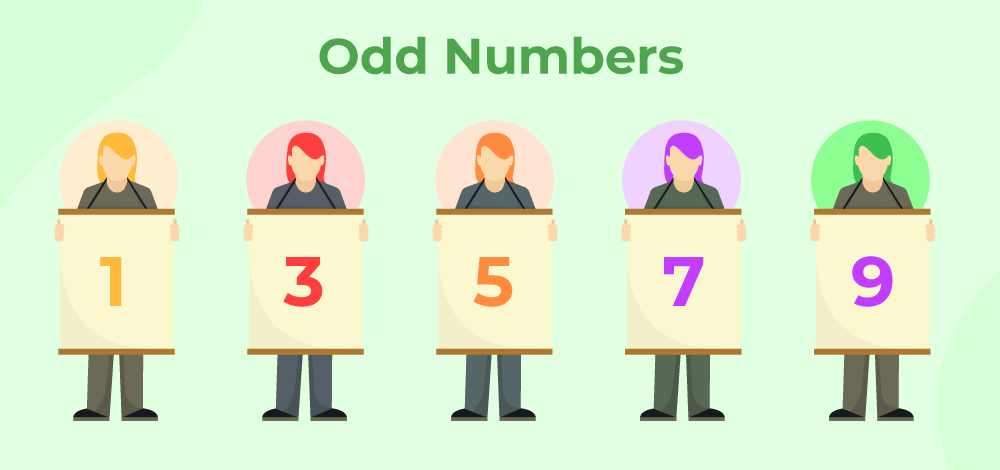### Even Natural Numbers

The natural numbers that are multiples of 2 i.e. completely divisible by 2 are known as Even Natural Numbers. Examples include 2,4, 18, 20, etc. Hence, the set of Even Natural Numbers can be represented by {2,4,6,8….}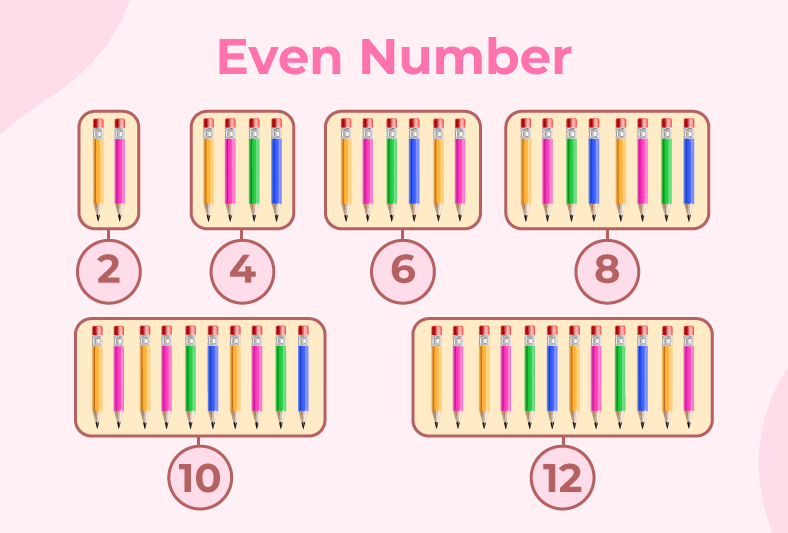### Smallest Natural Number

As natural number starts from 1 and goes till ∞ thus the smallest natural number is 1. While whole numbers generate from 0, therefore, the smallest whole number is 0.

## Natural Numbers from 1 to 100

As Natural Numbers are also called counting numbers, thus natural numbers from 1 to 100 are:

1, 2, 3, 4, 5, 6, 7, 8, 9, 10, 11, 12, 13, 14, 15, 16, 17, 18, 19, 20, 21, 22, 23, 24, 25, 26, 27, 28, 29, 30, 31, 32, 33, 34, 35, 36, 37, 38, 39, 40, 41, 42, 43, 44, 45, 46, 47, 48, 49, 50, 51, 52, 53, 54, 55, 56, 57, 58, 59, 60, 61, 62, 63, 64, 65, 66, 67, 68, 69, 70, 71, 72, 73, 74, 75, 76, 77, 78, 79, 80, 81, 82, 83, 84, 85, 86, 87, 88, 89, 90, 91, 92, 93, 94, 95, 96, 97, 98, 99, 100.

### Does 0 belong to Natural numbers?

The question is often asked if 0 is a natural number. To answer this question, we need to understand that natural numbers are counting numbers that start from 1 and go till ∞ and every successor is greater than its predecessor. Thus, 0 is not a natural number. The number 0 precisely belongs to the Whole number. 0 is also a part of Integers and is represented on the number line. However, even on the number line, every integer from +1 and its right side belongs to Natural numbers.

## Natural Numbers and Whole Numbers

The set of whole numbers is identical to the set of natural numbers, with the exception that it includes a 0 as an extra number. In mathematics, the set of whole integers is expressed as 0, 1, 2, 3,… The letter W stands for it. It is clear from the definitions that any natural number is a whole number. Furthermore, all whole numbers other than 0 are natural numbers. Below are the representation of the sets of natural numbers and whole numbers in roaster form,

W = {0, 1, 2, 3, 4, 5, …}

VS

N = {1, 2, 3, 4, 5, …}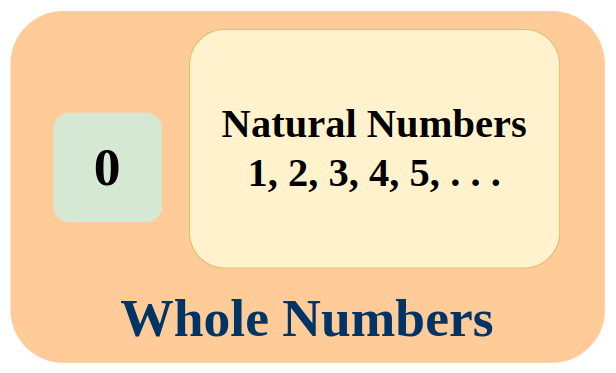### Difference Between Natural Numbers and Whole Numbers

Natural numbers, such as 1, 2, 3, 4, and so on, are all positive numbers. They’re the numbers used for counting, and they go on indefinitely. The entire numbers, on the other hand, are all natural numbers, excluding zero, such as 1, 2, 3, 4, and so on. All whole numbers and their negative counterparts are considered integers. -4, -3, -2, -1, 0,1, 2, 3, 4, and so on are some examples. The table below explains the difference between natural numbers and whole numbers,

## Natural Numbers on a Number Line

On the number line, the set of natural numbers and whole numbers is shown below. Natural numbers are represented by all positive integers or integers on the right-hand side of 0, whereas whole numbers are represented by all positive integers plus zero. The below diagram shows natural numbers and whole numbers on the number line.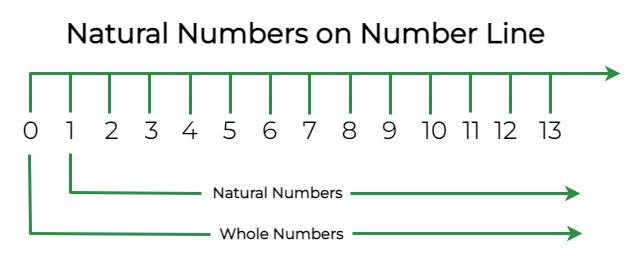## Properties of Natural Numbers

The properties of natural numbers have been derived from the properties of numbers. The four operations on natural numbers, addition, subtraction, multiplication, and division, resulting in four main characteristics of natural numbers, which are illustrated below:

• Closure property
• Commutative property
• Associative property
• Distributive property

### Closure Property

When two or more natural numbers are added and multiplied, the result is always a natural number. Addition Closure Property is a + b = c i.e. 3 + 2 = 5, 9 + 8 = 17. The sum of natural numbers is always a natural number, as this demonstrates. Multiplication Closure Property is ab = c i.e., 2 × 4 = 8, 7 × 8 = 56, etc. This demonstrates that a natural number is always the product of two natural numbers.

Note: Natural numbers may not obey the closure property when it comes to subtraction and division, which implies that subtracting or dividing two natural numbers may not result in a natural number.

### Associative Property

When adding and multiplying natural integers, the associative condition is true, i.e. a +(b + c) = (a + b) + c and a(b × c) = (a × b) c. Associative Property of Addition is a + (b + c) = (a + b) + c i.e; 1 + (3 + 5) = 1 + 8 = 9 and the same result is obtained in (1 + 3) + 5 = 4 + 5 = 9. Associative Property of Multiplication is a × (b × c) = (a × b) × c i.e; 2 × (2 × 1) = 2 × 2 = 4 and the same result is obtained in (a × b) × c = (2 × 2) × 1 = 4 × 1 = 4. Let’s brief both parts of the associative property of natural numbers,

• Associative Property of Addition: a + (b + c) = (a + b) + c
• Associative Property of Multiplication: a × (b × c) = (a × b) × c

Note: The associative property, on the other hand, does not hold true for natural number subtraction and division.

### Commutative Property

Even if the sequence of the numbers is changed, the sum or product of two natural numbers stays the same. The Commutative Property of N says that a + b = b + a and ab= ba for any a, b ∈ N. Let’s take a look at the commutative property of addition and the commutative property of multiplication for natural numbers,

• Commutative Property of Addition: a + b = b + a ⇒ Example: 4 + 5 = 9 and b + a = 5 + 4 = 9.
• Commutative Property of Multiplication: a × b = b × a ⇒ Example: 3 × 2 = 6 and 2 × 3 = 6.

### Distributive Property

The Distributive Property for natural numbers has two types, the distributive law of multiplication over addition and the distributive law of multiplication over subtraction. If we are given a(b + c), then a can be distributed between b and c and becomes (ab + ac). Similarly, a(b – c) can become (ab – ac).

• Distributive law of multiplication over addition: a(b + c) = ab + ac.
• Distributive law of multiplication over multiplication: a(b – c) = ab – ac.

Note: As 0 is the identity of addition but a set of natural numbers does not contain the element 0, thus there is no additive identity for the set of natural numbers. The set of Natural Numbers only contains the multiplicative identity i.e., 1.

Note: Also, for the inverse of the set of natural numbers for addition and multiplication. As there are only positive Integers in natural numbers, thus set does not contain either additive or multiplicative inverse.

## Operations on Natural Number

We can add, subtract, multiply, and divide the natural numbers together but the result in the subtraction and division is not always a natural number as natural numbers are not closed under the subtraction and division operation. Let’s understand the operations between natural numbers in much detail.

The addition operation on natural numbers combines two or more numbers to find their total. The symbol used to represent addition is “+”. For example, the sum of 3 and 4 is written as 3 + 4 = 7, 11 + 17 = 28, 21 + 57 = 78, 34 + 111 = 145, etc.

### Subtraction of Natural Number

The subtraction operation on natural numbers finds the difference between two natural numbers it can output a natural number and non-natural numbers (i.e., integers) as natural numbers are not closed under subtraction. The symbol used to represent subtraction is “-“. For example, the difference between 5 and 3 is written as 5 – 3 = 2, 11 – 5 = 6, 17 – 21 = -4, 24 – 1 = 23, etc.

### Multiplication of Natural Number

The multiplication operation of natural numbers finds the value of repeated addition i.e., 2 multiplied by 4 mean addition of 2 to itself four times or addition of 4 to itself two times. The symbol used to represent multiplication is “×” or “*”. For example, the product of 3 and 4 is written as 3 × 4 = 12, 7 × 11 = 77, 12 × 9 = 108, 24 × 5 = 120, etc.

### Division of Natural Number

The division operation of natural numbers divides the number into equal parts i.e., if we divide 12 by 3, then can get 4 as each equal part. The symbol used to represent division is “÷” or “/”. For example, the quotient of 12 divided by 3 is written as 12 ÷ 3 = 4, 22 ÷ 11 = 2, 48 ÷ 12 = 4, etc.

If the number can’t be divided into equal parts i.e., divide 12 by 5, as we can only divide 12 into parts of unit 5 and the rest i.e., 2 is called the remainder. Thus, Natural Numbers are not closed under the operation of division. For some more examples, when 22 is divided by 7, we get 3 as quotient and 1 as remaining i.e., 22 =  7×3 +1, 43 = 8×5 + 3, etc.

Other important operations defined for the natural number are as follows:

### Exponentiation of Natural Numbers

Exponentiation is the operation of raising a number to a certain power. The symbol used to represent exponentiation is “^”. For example, 2 raised to the power of 3 is written as 2^3 or 23 = 8.

### Square Root of Natural Numbers

The square root of a number is the value that, when multiplied by itself, gives the original number. The symbol used to represent the square root is “√”. For example, the square root of 25 is written as √25 = 5.

### Factorial of Natural Numbers

The factorial of a natural number is the product of all positive integers up to and including that number. The symbol used to represent factorial is “!”. For example, 5 factorial is written as 5! = 5 × 4 × 3 × 2 × 1 = 120.

## Sum of Natural Numbers

Natural numbers are 1, 2, 3, 4,

### Sum of First n Natural Numbers

The sum of the first n natural numbers is given by

S = n(n+1)/2

Where n is the number of terms taken into consideration.

Example: Find the sum of the first 50 Natural Numbers.

Solution:

The sum of the first n natural numbers can be found using the formula:

S = n(n + 1)/2

Using n = 50, we get:

S = 50(50 + 1)/2 = 1275

Therefore, the sum of the first 50 natural numbers is 1275.

### Mean of First n Natural Numbers

As mean is defined as the ratio of the sum of observations to the number of total observations, thus mean for the first n terms of natural number can be calculated using the formula:

Mean = S/n = (n+1)/2

Where,

• S is the sum of all observations, and
• n is the number of terms taken into consideration.

Example: Find the mean of the first 20 natural numbers.

Solution:

The sum of the first n natural numbers can be found using the formula:

S = n(n + 1)/2

and mean can be calculated using formula

Mean = S/n

Using n = 20, we get:

S = 20(20 + 1)/2 = 210

and Mean = S/n = 210/20 = 10.5

Therefore, the mean of the first 20 natural numbers is 10.5.

### Sum of Square of First n Natural Numbers

The sum of the square of the first n natural numbers is given as follows:

S = n(n + 1)(2n + 1)/6

Where n is the number of taken into consideration.

Example: Find the sum of the Square of the first 12 natural numbers.

Solution:

As we need to find the sum of first 12 natural number, use n = 12 in the formula

S = n(n + 1)(2n + 1)/6

⇒ S = 12(12 + 1)(2×12 + 1)/6

⇒ S = 12×13×25/6

⇒ S = 2×13×25

⇒ S = 650

Thus, sum of square of first 12 natural numbers is 650.

## Solved Examples of Natural Numbers

Example 1: Identify the natural numbers among the given numbers:

23, 98, 0, -98, 12.7, 11/7, 3.

Since negative numbers, 0, decimals, and fractions are not a part of natural numbers. Therefore, 0, -98, 12.7, and 11/7 are not natural numbers.

Therefore, natural numbers are 23, 98, and 3.

Example 2: Prove the distributive law of multiplication over addition with an example.

Distributive law of multiplication over addition states: a(b + c) = ab + ac.

For example, 4(10 + 20), here 4, 10, and 20 are all natural numbers and hence must follow the distributive law. Therefore,

4(10 + 20) = 4 × 10 + 4 × 20

4 × 30 = 40 + 80

120 = 120

Hence, proved.

Example 3: Prove the distributive law of multiplication over subtraction with an example.

Distributive law of multiplication over addition states: a(b – c) = ab – ac.

For example, 7(3 – 6), here 7, 3, and 6 are all natural numbers and hence must follow the distributive law. Therefore,

7(3 – 6) = 7 × 3 – 7 × 6

7 × -3 = 21 + 42

-21 = -21

Hence, proved.

Example 4: List the first 10 natural numbers.

1, 2, 3, 4, 5, 6, 7, 8, 9, and 10 are the first ten natural numbers.

Example 5: Identify the non-natural numbers among the given numbers:

45, 9, 10, -8, 1.17, 98/3, 33/11.

Since negative numbers, 0, decimals, and fractions are not a part of natural numbers. Therefore, -8, 1.17, 98/3 are non-natural numbers. Note that 33/11 can be simplified to 3 and 3 is a natural number.

## FAQs on Natural Numbers

### Q1: What is a Natural Number in Math?

The number used for counting such as 1, 2, 3, 4, 5, . . . so on to infinity, are called natural numbers and any element from this collection is a natural number.

### Q2: Is 0 a Natural Number?

No, 0 is not a part of natural numbers. 0 is a part of whole numbers, and this is the major difference between whole numbers and natural numbers.

### Q3: Which is the Smallest Natural Number?

The smallest natural number is 1. Natural numbers begin at 1 and go up to infinity. Therefore, the smallest natural number is 1.

### Q4: How many Natural Numbers are there?

There are uncountabley infinite natural numbers.

### Q5: Which Natural Number has no Predecessor?

As 1 is the first and smallest natural number, thus it doesn’t have any predecessor in the set of natural numbers.

### Q6: Which Natural number has no Successor?

As there are infinitely many natural number so we don’t know the last natural number. Thus there is no such natural number which do not have successor or in other words we can say each natural number always has a successor.

### Q7: Are Natural Numbers Whole Numbers?

Yes, as set of natural number is subset of the whole number or we can say whole number are natural number with 0. Thus all the natral number are whole number.

### Q8: Every Whole Number is a Natural Number. True or False?

False. Every whole number is not a natural number as 0 is involved in whole numbers but not in natural numbers. Therefore, the assertion is wrong.

### Q9: How many Natural Numbers lie between 1 and 100 (not including both)?

As natural number are 1, 2, 3, 4, 5, . . . so on,

Thus, there are exactly 100 natural number till number 100, but as we don’t have to include the 1 and 100.

Thus, there are 100 – 2 = 98, natural number in between 1 and 100.

### Q10: What is the Sum of First n Natural Numbers?

The formula for the sum of first n natural numbers is:

S = n (n + 1)/2

Here, n is the number of terms.

### Q11: What is the Sum of the First 10 Natural Numbers?

1, 2, 3, 4, 5, 6, 7, 8, 9, and 10 are the first ten natural numbers. Therefore, the sum of the first 10 natural numbers will be 1 + 2 + 3 + 4 + 5 + 6 + 7 + 8 + 9 + 10 = 55.

### Q12: Find the Mean of First n Natural Numbers.

The mean of first n natural numbers is,

(n + 1)/2

Where n is the number of terms.